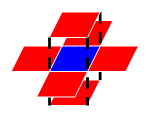# 1091 Acute Stroke

One important factor to identify acute stroke (急性脑卒中) is the volume of the stroke core. Given the results of image analysis in which the core regions are identified in each MRI slice, your job is to calculate the volume of the stroke core.

### Input Specification:

Each input file contains one test case. For each case, the first line contains 4 positive integers: M, N, L and T, where M and N are the sizes of each slice (i.e. pixels of a slice are in an M×N matrix, and the maximum resolution is 1286 by 128); L (≤) is the number of slices of a brain; and T is the integer threshold (i.e. if the volume of a connected core is less than T, then that core must not be counted).

Then L slices are given. Each slice is represented by an M×N matrix of 0‘s and 1‘s, where 1 represents a pixel of stroke, and 0 means normal. Since the thickness of a slice is a constant, we only have to count the number of 1‘s to obtain the volume. However, there might be several separated core regions in a brain, and only those with their volumes no less than T are counted. Two pixels are connected and hence belong to the same region if they share a common side, as shown by Figure 1 where all the 6 red pixels are connected to the blue one.Figure 1

### Output Specification:

For each case, output in a line the total volume of the stroke core.

### Sample Input:

``````3 4 5 2
1 1 1 1
1 1 1 1
1 1 1 1
0 0 1 1
0 0 1 1
0 0 1 1
1 0 1 1
0 1 0 0
0 0 0 0
1 0 1 1
0 0 0 0
0 0 0 0
0 0 0 1
0 0 0 1
1 0 0 0
``````

### Sample Output:

``26``

Code:

```#include<iostream>
#include<vector>
#include<queue>

using namespace std;

int m, n, l, t;
int count = 0, rang = 0, total = 0;
vector<vector<vector<int> > > matrix;
vector<vector<vector<int> > > visited;
vector<int> dir = {1, 0, 0, -1, 0, 0, 1, 0};

bool inMatrix(int i, int j, int k) {
if (i >= 0 && i < l && j >= 0 && j < m && k >= 0 && k < n)
if (matrix[i][j][k] == 1 && visited[i][j][k] == 0) return true;
return false;
}

void BFS(int x, int y, int z) {
visited[x][y][z] = 1;
count++;
for (int i = 0; i < 6; ++i) {
if (inMatrix(x+dir[i], y+dir[i+1], z+dir[i+2]))
BFS(x+dir[i], y+dir[i+1], z+dir[i+2]);
}
}

int main() {
cin >> m >> n >> l >> t;

matrix = vector<vector<vector<int> > >(l, vector<vector<int> >(m, vector<int>(n, 0)));
visited = vector<vector<vector<int> > >(l, vector<vector<int> >(m, vector<int>(n, 0)));

for (int i = 0; i < l; ++i) {
for (int j = 0; j < m; ++j) {
for (int k = 0; k < n; ++k) {
int p;
cin >> p;
matrix[i][j][k] = p;
}
}
}
for (int i = 0; i < l; ++i) {
for (int j = 0; j < m; ++j) {
for (int k = 0; k < n; ++k) {
count = 0;
if (visited[i][j][k] == 0 && matrix[i][j][k] == 1)
BFS(i, j, k);
if (count >= t) total += count;
}
}
}

cout << total << endl;

return 0;
}
```

1091 Acute Stroke

(0)
(0)